Mathematics
Easy

Question

# Suppose y varies inversely as the square of x. If x is multiplied by 4 , which of the following is true for the value of y?

## It is multiplied by 4 It is multiplied by 16It is multiplied by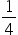It is multiplied by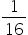Hint:

## The correct answer is: It is multiplied by### We have to find the value of y  when y varies inversely to the square of x.We have, y =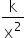If x becomes 4x, then: y =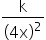=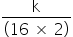So, y is multiplied by.Hence, the correct option is D.

Instead of taking y= k/x², we could also have taken yx² = k, where k is a constant.

### Related Questions to study#### With Turito Foundation.#### Get an Expert Advice From Turito.